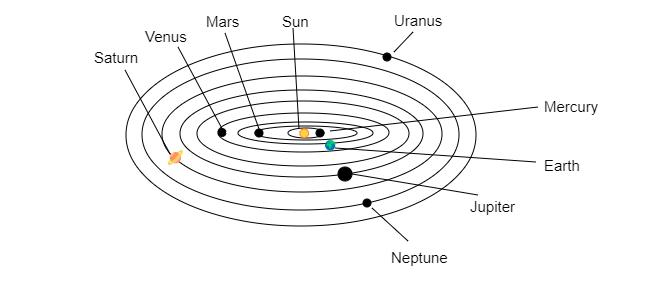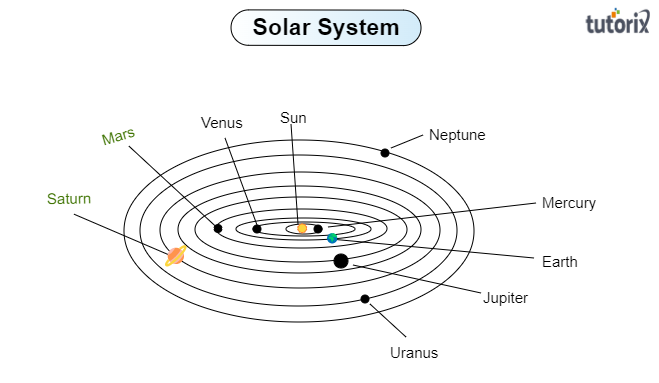# Boojho made the following sketch (Fig. 17.29) of the solar system. Is the sketch correct? If not, correct it."

Given:

Boojho made the following sketch $(Fig.\ 17.29)$ of the solar system.

To do:

We have to check whether the sketch made by Bhoojo is correct. If the sketch is not correct then we have to correct it.

Solution:

Let us observe the given sketch of the solar system made by Bhoojo.

Observation:

Let us check the planets placed in the correct and incorrect positions in the given sketch of the solar system-

Correct position-

Sun, Earth, Mercury, Saturn, and Jupiter are placed in the correct position in the given sketch.

Incorrect position-

Boojho has interchanged the position of Mars and Venus and also Neptune with Uranus.

On observing the given sketch of the solar system made by Bhoojo we find that the sketch is not correct. Let us draw the correct sketch of the solar system-

Correct sketch of the solar system:

The correct sketch of the solar system is as below-